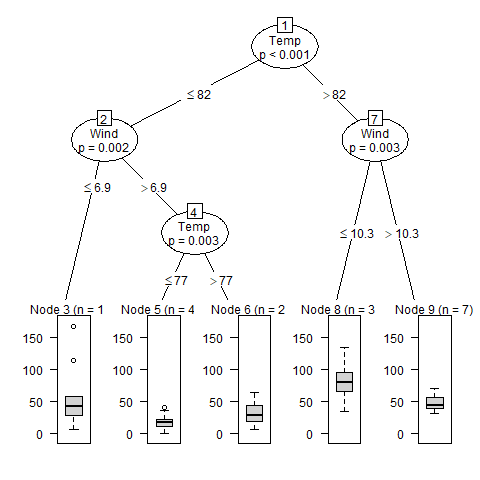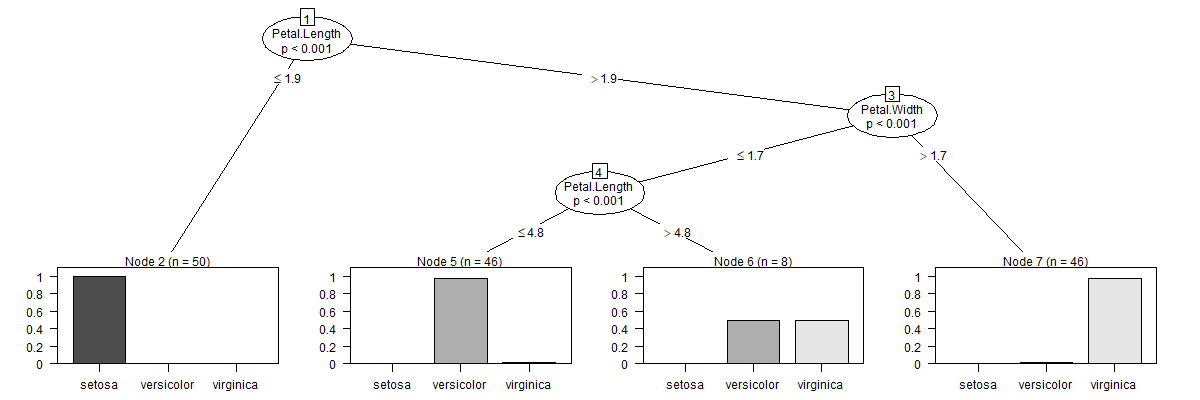Related Articles
Conditional Inference Trees in R Programming
• Last Updated : 10 Jul, 2020

Conditional Inference Trees is a non-parametric class of decision trees and is also known as unbiased recursive partitioning. It is a recursive partitioning approach for continuous and multivariate response variables in a conditional inference framework. To perform this approach in R Programming, `ctree()` function is used and requires `partykit` package. In this article, let’s learn about conditional inference trees, syntax, and its implementation with the help of examples.

#### Conditional Inference Trees

Conditional Inference Trees is a different kind of decision tree that uses recursive partitioning of dependent variables based on the value of correlations. It avoids biasing just like other algorithms of classification and regression in machine learning. Thus, avoiding vulnerability to the errors making it more flexible for the problems in the data. Conditional inference trees use a significance test which is a permutation test that selects covariate to split and recurse the variable. The p-value is calculated in this test. The significance test is executed at each start of the algorithm. This algorithm is not good for data with missing values for learning.
Algorithm:

1. Test the global null hypothesis between random input and response variables and select the input variable with the highest p-value with response variable.
2. Perform binary split on the selected input variable.
3. Recursively perform step 1 and 2.

#### How Conditional Inference Trees differs from Decision Trees?

Conditional Inference Trees is a tree-based classification algorithm. It is similar to the decision trees as `ctree()` also performs recursively partitioning of data just like decision trees. The only procedure that makes conditional inference trees different from decision trees is that conditional inference trees use a significance test to select input variables rather than selecting the variable that maximizes the information measure. For example, the Gini coefficient is used in traditional decision trees to select the variable that maximizes the information measure.

#### Implementation in R

Syntax:
ctree(formula, data)

Parameters:
formula: represents formula on the basis of which model is to be fit
data: represents dataframe containing the variables in the model

Example 1:

In this example, let’s use the regression approach of Condition Inference trees on the air quality dataset which is present in the R base package. After the execution, different levels of ozone will be determined based on different environmental conditions. This helps in learning the different behavior of ozone value in different environmental conditions.

Step 1: Installing the required packages.

 `# Install the required ``# Package for function``install.packages``(``"partykit"``)`

Step 2: Loading the required package.

 `# Load the library``library``(partykit)`

Step 3: Creating regression model of Condition inference tree.

 `air <- ``subset``(airquality, !``is.na``(Ozone))``airConInfTree <- ``ctree``(Ozone ~ ., ``                       ``data = air)`

Step 4: Print regression model.

 `# Print model``print``(airConInfTree)`

Output:

```Model formula:
Ozone ~ Solar.R + Wind + Temp + Month + Day

Fitted party:
 root
|    Temp <= 82
|   |    Wind  6.9
|   |   |    Temp  77: 31.143 (n = 21, err = 4620.6)
|    Temp > 82
|   |    Wind  10.3: 48.714 (n = 7, err = 1183.4)

Number of inner nodes:    4
Number of terminal nodes: 5
```

Step 4: Plotting the graph.

 `# Output to be present as PNG file``png``(file = ``"conditionalRegression.png"``)`` ` `# Plotting graph``plot``(airConInfTree)`` ` `# Save the file``dev.off``()`

Output:Explanation:
After executing, the above code produces a graph of conditional inference tree that shows the ozone value in the form of a box plot in each node in different environmental conditions. As in the above output image, Node 5 shows the minimum ozone value. Further, learning the behavior shows Temp6.9 shows the least ozone value in air quality.

Example 2:

In this example, let’s use the classification approach of Condition Inference trees on the iris dataset present in the R base package. After executing the code, different species of iris plants will be determined on the basis of petal length and width.

Step 1: Installing the required packages.

 `# Install the required ``# Package for function``install.packages``(``"partykit"``)`

Step 2: Loading the required package.

 `# Load the library``library``(partykit)`

Step 3: Creating classification model of Condition inference tree

 `irisConInfTree <- ``ctree``(Species ~ ., ``                        ``data = iris)`

Step 4: Print classification model

 `# Print model``print``(irisConInfTree)`

Output:

```Model formula:
Species ~ Sepal.Length + Sepal.Width + Petal.Length + Petal.Width

Fitted party:
 root
|    Petal.Length  1.9
|   |    Petal.Width <= 1.7
|   |   |    Petal.Length  4.8: versicolor (n = 8, err = 50.0%)
|   |    Petal.Width > 1.7: virginica (n = 46, err = 2.2%)

Number of inner nodes:    3
Number of terminal nodes: 4
```

Step 4: Plotting the graph

 `# Output to be present as PNG file``png``(file = ``"conditionalClassification.png"``,``width = 1200, height = 400)`` ` `# Plotting graph``plot``(irisConInfTree)`` ` `# Save the file``dev.off``()`

Output:Explanation:
After executing the above code, species of iris plants are classified based on petal length and width. As in above graph, setosa species have petal length <= 1.9.

Attention reader! Don’t stop learning now. Get hold of all the important DSA concepts with the DSA Self Paced Course at a student-friendly price and become industry ready.

My Personal Notes arrow_drop_up